## LetsPlayMaths.Com

WELCOME TO THE WORLD OF MATHEMATICS

# Class 5 Decimal Number

Decimal Numbers

Conversion of Decimal Numbers into Fractions

Conversion of Fractions into Decimal Numbers

Rounding Off Decimal Numbers

Addition of Decimal Numbers

Subtraction of Decimal Numbers

Multiplication of Decimal Numbers by 10,100,1000,etc.

Multiplication of Decimal Numbers by Whole Number

Multiplication of Decimal Number by a Decimal Number

Multiplication Properties of Decimal Numbers

Division of Decimal Numbers

Decimal Numbers Test

Decimal Numbers Worksheet

Answer Sheet

## Decimal Numbers

In class IV, we have learnt how fractions with denominators like 10, 100, 1000 can be converted into decimal numbers. For example, 2/10 , 15/100 can be written as 0.2 and 0.15 respectively.

A decimal number has two parts, one part is whole part and the other part is decimal part. Both the parts are separated by a dot. Let’s see one example.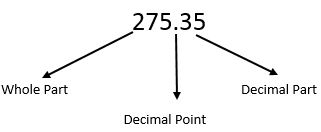## Conversion of Decimal Numbers into Fractions

To convert a decimal number into a fraction, we must follow the below mentioned steps.

1. Write all the digits of the decimal number as numerator of the fraction without considering the
decimal point.
2. Count the number of digits present to the right of the decimal point and write as many zeros
after 1 in the denominator of the fraction.

Example 1. Convert 25.321 to a fraction.

Solution. 25.321 is the decimal number.
25321 should be the numerator of the fraction.
Number of digits present to the right of the decimal point = 3
Denominator of the fraction should be 1000
So, the fraction will be 25321/1000.

## Conversion of Fractions into Decimal Numbers

1. If the denominator is not 10, 100, 1000, etc. then Convert the denominator into 10, 100, 1000, etc.
2. Count the number of zeros in the denominator after 1.
3. Count the numerator’s digit starting from right till it is equal to the number of zeros present in
the denominator and place a decimal point towards the left of the digit.

Example 1. Convert 275/100 into decimal numbers.

Solution. Number of zeros present in the denominator = 2
If we count the numerator’s digit starting from the right for 2 times, then we will reach at 7. We must
put a decimal point between 2 and 7 digits of the numerator.
So. The decimal number is equal to 2.75.

Example 2. Convert 121/25 into decimal numbers.

Solution. We must convert denominator 25 to 100, for that we must multiply 4 with both denominator and numerator.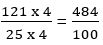So, the decimal number is equal to 4.84.

## Rounding Off Decimal Numbers

We have already learned how to round off numbers to the nearest thousands, hundreds and tens. We will use same methods to round off decimal numbers.

Round Off to the Nearest Tenth
To round off to the nearest tenths, we must compare the digits at the hundredths place with 5. If the digit is less than 5 then we ignore all the digits in the places to the right of the tenths place. If the digit is 5 or greater than 5 in the hundredths place, then we round off the decimal number to the next tenths place and ignore all the remaining digits to the right of tenths place.

Example 1. Round off 25.437 to the nearest tenths place.

Solution. Digits in hundredths place is 3, that is 3 < 5. So, the number is rounded off to 25.4.

Example 2. Round off 56.273 to the nearest tenths place.

Solution. Digits in hundredths place is 7, that is 7 > 5. So, the number is rounded off to 56.3.

Round Off to the Nearest Hundredth
To round off to the nearest hundredths, we must compare the digits at the thousandths place with 5. If the digit is less than 5 then we ignore all the digits in the places to the right of the hundredths place. If the digit is 5 or greater than 5 in the thousandths place, then we round off the decimal number to the next hundredths place and ignore all the remaining digits to the right of hundredths place.

Example 1. Round off 2.564 to nearest hundredths place.

Solution. Digits in thousandths place is 4, that is 4 < 5. So, the number is rounded off to 2.56.

Example 2. Round off 72.567 to nearest hundredths place.

Solution. Digits in thousandths place is 7, and 7 > 5. So, the number is rounded off to 72.57.

## Addition of Decimal Numbers

Decimal number addition is like our normal addition. But, here we must follow certain rules that are mentioned below.

1. First convert all the decimals to like decimals.
2. Write the decimal numbers in column format. Unit place should come in one column, tens place should come
in one column, hundred place should come in one column, thousand place should come in one column and so on.
Decimal point should come in one column, tenth come in one column, hundredth come in one column and so on.
3. Do the normal addition keeping decimal point between whole and decimal number.

Example 1. Add 5.32, 6.315 and 12.04.

Solution.Arrange the all decimal numbers in tabular format as shown below.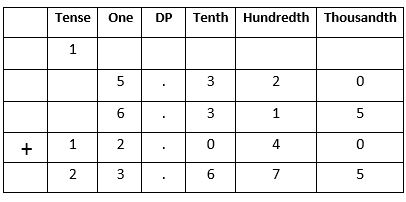So, the answer is 23.675.

## Subtraction of Decimal Numbers

Decimal number subtraction is like our normal subtraction. But, here we must follow certain rules that are mentioned below.

1. First convert all the decimals to like decimals.
2. Write the decimal numbers in column format. Unit place should come in one column, tens place should
come in one column, hundred place should come in one column, thousand place should come in one column
and so on. Decimal point should come in one column, tenth come in one column, hundredth come in one
column and so on. 3. Do the normal subtraction keeping decimal point between whole and decimal number.

Example 1. Subtract 45.29 from 276.671.

Solution.Arrange all the decimal numbers in tabular format as shown below.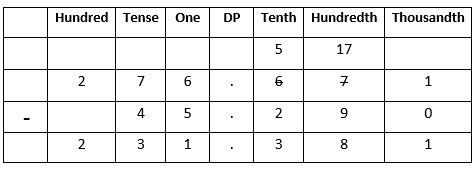So, the answer is 231.381.

## Multiplication of Decimal Numbers by 10,100,1000,etc.

When we multiply a decimal number by 10, 100, 1000, etc. then the result will also be a decimal number. But, the decimal point will be shifted to the right by as many places as there are zeros in the multiplier after 1.

Example 1. Multiply 24.34527 by 1000.

Solution. Number of zeros in 1000 = 3
So, the decimal point will move 3 places to the right.
24.34527 x 1000 = 24345.27

## Multiplication of Decimal Numbers by Whole Number

When we multiply a decimal number with a whole number, the result will have as many digits after the decimal point as the multiplicand.

Example 1. Multiply 25.24 by 5.

Solution.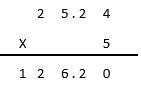So, the answer is 126.20.

Example 2. Multiply 132.452 by 12.

Solution.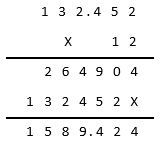So, the answer is 1589.424.

## Multiplication of Decimal Number by a Decimal Number

When we multiply both decimal numbers, the result will have as many digits after the decimal point as the sum of the number of digits after the decimal points of the multiplicand and the multiplier.

Example 1. Multiply 1.2 by 0.8.

Solution.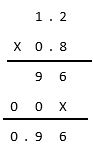So, the answer is 0.96

Example 2. Multiply 23.432 by 12.34.

Solution.So, the answer is 289.15088.

## Multiplication Properties of Decimal Numbers

1. Product of a decimal number and zero is zero.

2. Product of a decimal number and 1 is decimal number itself.

3. Changing the order of multiplication of a decimal number with a whole number or a decimal number does not

change the results.

## Division of Decimal Numbers

In class IV, we have learnt division of decimal numbers by 10, 100, 1000 etc. Here, we will learn division of decimal number by whole number and decimal number.

Division of Decimal Number by 10, 100, 1000, etc.
When we division a decimal number by 10, 100, 1000, etc., the decimal point is shifted to left depending on the number of zeros present. For example, if a decimal number is divided by 10 then the result will remain same, but the decimal point will be shifted to the left by one place. Similarly, if a decimal number is divided by 100 then the decimal point will be shifted to the left by two places.

Example 1. Divide 8.7 by 10.

Solution.Example 2. Divide 134.26 by 100.

Solution.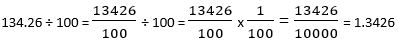Division of Decimal Number by Whole Number
When we divide decimal number by a whole number, as soon as the tenths digit comes down from the dividend, a decimal point is placed in the quotient. Let’s see some examples.

Example 1. Divide 27.5 by 5.

Solution.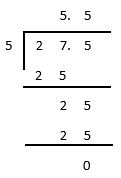So, the answer is 5.5.

Example 2. Divide 64.1 by 2.

Solution.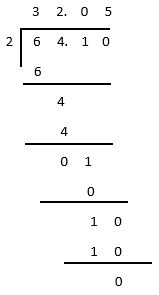So, the answer is 32.05.

Division of Decimal Number by Decimal Number
When we divide a decimal number by another decimal number, first we must convert the divisor into a whole number and then divide the dividend decimal number by the whole number. Let’s have a look at some examples.

Example 1. Divide 230.22 by 0.3.

Solution. First, we must convert the divisor 0.3 into a whole number.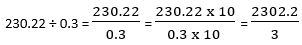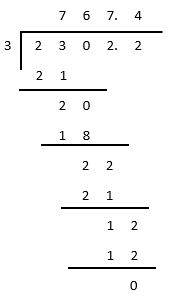So, the answer is 767.4.

Example 2. Divide 41.535 by 9.23.
Solution. First, we must convert the divisor 9.23 into a whole number.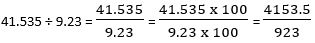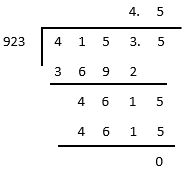So, the answer is 4.5.

## Decimal Number Test

Decimal Number Test - 1

Decimal Number Test - 2

## Class-5 Decimal Number Worksheet

Decimal Number Worksheet - 1

Decimal Number Worksheet - 2

Decimal Number Worksheet - 3

Decimal Number Worksheet - 4

Decimal Number Worksheet - 5

## Answer Sheet

Decimals-AnswerDownload the pdf

Copyright © 2021 LetsPlayMaths.com. All Rights Reserved.